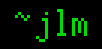## 24-Sep-2005

### How big is an Orbital?

Filed under: math — jlm @ 06:38

In science fiction, it’s fairly common to use centrifugal force to simulate gravity in space. This is usually used for ships or space stations, but Larry Niven took it to an extreme with his famous Ringworld, which circles a star at orbital distance and spins enough faster than orbital speed to provide Earth-equivalent “gravity” against its inside. Iain Banks thought this was too extreme even for fantastically-powerful civilizations, so toned it down a bit by having a ring that revolves once per day and provides 1 g from its spin. (Niven’s Ringworld needed “shadow squares” between the ring and the star to provide night.) Banks calls these “Orbitals”, places them in planetary orbits, and implies that they’re of planetary dimensions. But, how big is an Orbital really?

Well, we want a period of about an Earth day (d), and centripetal acceleration of about an Earth gravity (g). Consider a point x on the Orbital at angle θ. x = r î cos θ + r ĵ sin θ. To provide an Earth day, the Orbital revolves through 2π radians in 1 d, so dθ/dt = 2π/d. Acceleration is the second derivative of position, so a=d²x/dt²=(-r î cos θ-r ĵ sin θ)(4π²/d²). We want ∥a∥ to be 1 g, so ∥a∥ = r·(4π²/d²) = g, or r = g·(d²/4π²). To match Earth, g should be about 9.8 m/s² and d about 86400 s, which puts r at about 1.9×109 m.

That’s huge! (Though not Ringworld huge.) The radius of the Earth is only 6×106 m, and Jupiter is “only” 7×107. An Orbital is 300 times as wide as the Earth. (Though potentially much shorter.) But still not orbital distances– it’s only 1% of the size of Earth’s orbit.

Now, how strong does an Orbital have to be? Well, let’s consider a small section of the Orbital subtending an angle Δθ. This will have length Δl = r·Δθ. If the Orbital has a linear density σ, this section will have mass ΔM = σ·Δl and weight Δw = g·ΔM = gσr Δθ. If the tension is T, the internal force at the edge of the section will be T î sin ½Δθ + T ĵ cos ½Δθ. The i component of this force holds “up” half of the section’s weight, the other edge providing the other half. Using the small angle approximation for sine, we get ½Δw = T·½Δθ, or T = gσr. So the strength we need is T/σ = gr = 2×1010 m²/s². If the Orbital’s cross-sectional area is τ, then the internal tensile pressure is P=T/τ so T = P·τ. If its material density is δ, then σ=δ·τ, so T/σ = P·τ / δ·τ = P/δ, which tells us that the 2×1010 m²/s² is the ratio of tensile strength to density we need. This is 10000 times as strong as existing high-tension materials like Kevlar (stuff like steel and titanium and diamond isn’t particularly strong in the tension department) and still 600 times as strong as the supposed carbon nanotubes that they say may make a space elevator possible. And this is just enough for the structure to not tear itself apart by its own spin, much less support anything you want to have on the Orbital. I thought solar tides might be an issue, being about 9×1020 N for each Earth mass of the Orbital, but the spin tension blows that away at 6×1025 N per Earth mass. So, sadly, even through this is orders of magnitude more feasible than the Ringworld, it’s still never going to work.